## Breakdown in Non Uniform Fields:

In Breakdown in Non Uniform Fields, such as coaxial cylinders, point-plane and sphere-plane gaps, the applied field varies across the gap. Similarly, Townsend’s first ionization coefficient (α) also varies with the gap. Hence αd in Townsend’s criterion [refer to Eq. (2.22)] is rewritten by replacing αd by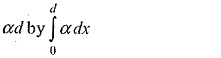Townsend’s criterion for breakdown now becomes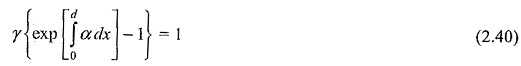Meek and Raether also discussed the non-uniform field breakdown process as applied to their Streamer theory, and the Meek’s equation [Eq. (2.27)] for the radial field at the head of an avalanche when it has crossed a distance x is modified as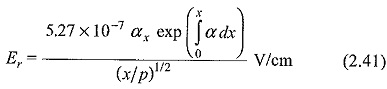where αx is the value of α at the head of the avalanche, and p is the gas pressure. The criterion for the formation of the streamer is reached when the space charge field Er approaches a value equal to the applied field at the head of the avalanche.

This equation has been successfully used for determining the corona onset voltages of many non-uniform geometries. However, the condition for the advancement of streamers has not been arrived at so far. Figures 2.16 to 2.18 show the breakdown characteristics for SF6 and SF6/N2 mixtures.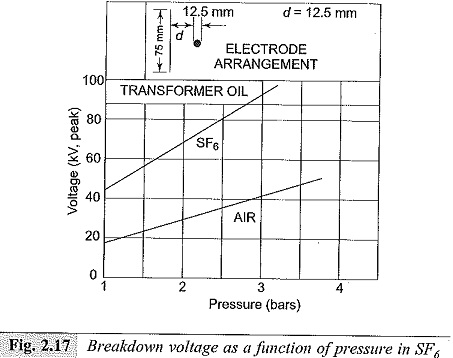From the practical engineering point of view, rod-rod gap and sphere-sphere gap are of great importance, as they are used for the measurement of high voltages and for the protection of electrical apparatus such as transformers. The breakdown characteristics of rod-rod gaps are shown in Fig. 2.19. From this figure it can be seen that the breakdown voltages are higher for negative polarity.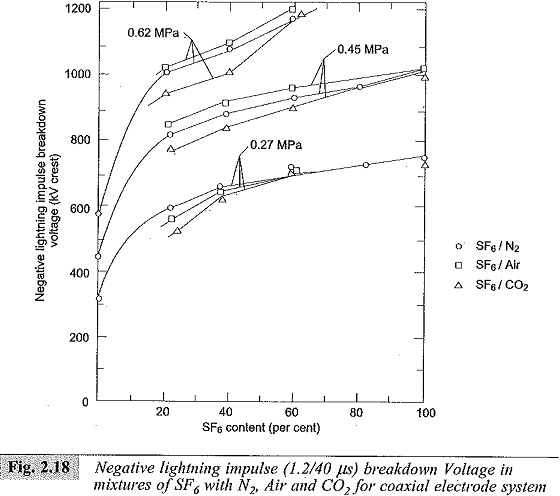The breakdown voltages were also observed to depend on humidity in air. In the case of rod gaps the field is non-uniform, while in the case of sphere gaps field is uniform, if the gap is small compared with the diameter. In the case of sphere gaps, the breakdown voltages do not depend on humidity and are also independent of the voltage waveform. The formative time lag is quite small (~0.5μs) even with 5% over-voltage. Hence sphere gaps are used for breakdown voltage (peak value) measurements.

Scroll to Top